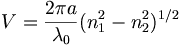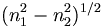# Optical Fiber Waveguides

A circular dielectric waveguide or fiber optic has an internal core that has a higher index of refraction than the cladding. At a certain diameter there is an angle that is less than the critical angle so there is total internal reflection.

These are referred to as step index fibers. The y axis refers to the index of refraction. The x axis is the distance from the center of the core. So the highest index of refraction (n1) is found in the core and there is a significant step in the index of refraction (n2) at the interface with the cladding.

In most practical waveguides the refractive indices of the core and cladding differ from each other by only a few percent.

The number of modes in a step-index circular waveguide is determined by the V parameter where$V= \frac {2\pi a} {\lambda_0} (n^2_1 - n^2_2)^{1/2}\,\!$

where$a\,\!$ is the core radius$(n^2_1 - n^2_2)^{1/2}\,\!$ is referred to as the numerical aperature, the larger this term the larger the number of modes.

The fiber can support only one mode when V < 2.405

For n1 = 1.48 and n2 = 1.46, the radius of the core a <2.7μm

There is current research to develop a core with a graded index of refraction that gradually decreases to equal that of the cladding. This is referred to as a graded index fiber.